## 详情信息展示

Table 1 Electrode reactions of electrolysis process of waste lead paste by hydrometallurgy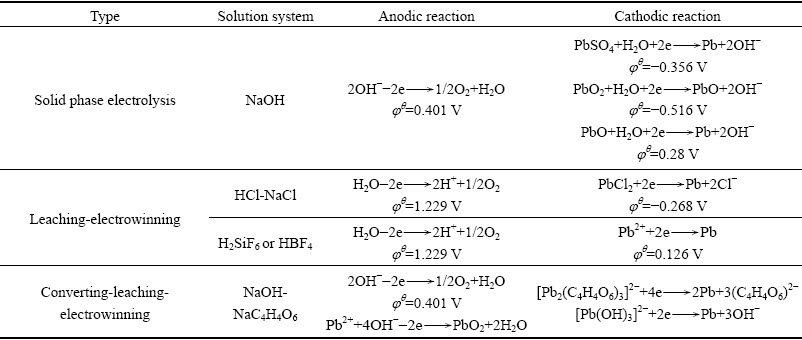4  废铅膏低温还原清洁炼铅的技术思路

4.1  新技术思路的产生

4.2  原料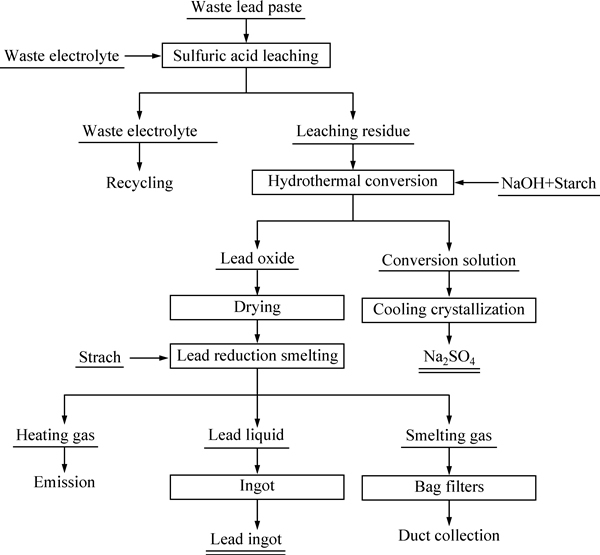Fig. 2  Flow sheet of lead clean smelting from waste lead paste at low temperature referred to aluminum metallurgy

Table 2  Main composition of waste lead paste (mass fraction, %)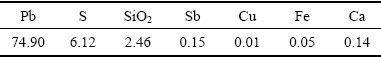Table 3  Proportion of lead phase in waste lead paste(mass fraction, %)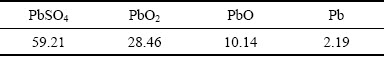4.3  废铅膏脱除杂质

Cu+2H2SO4+PbO2=CuSO4+PbSO4↓+2H2O       (1)

Fe+2H2SO4 +PbO2=FeSO4+PbSO4↓+2H2O        (2)

2Sb+4H2SO4 +3PbO2= (SbO)2SO4+3PbSO4↓+4H2O (3)

PbO+H2SO4=PbSO4↓+H2O                    (4)

Pb +2H2SO4+PbO2=2PbSO4↓+2H2O             (5)

Table 4  Proportion of lead phase in sulfuric acid leaching residue(mass fraction, %)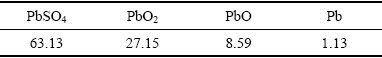4.4  水热还原双重转化

PbSO4+2NaOH=PbO+Na2SO4+H2O              (6)

(C6H10O5)n+12PbO2=12PbO+6CO2↑+5H2O        (7)

Table 5  Proportion of lead phase in lead oxide (mass fraction, %)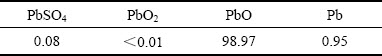4.5  低温还原清洁炼铅

12PbO+(C6H10O5)n=12Pb+6CO2↑+5H2O          (8)